# 详解哈希数据结构，手写哈希表

2021-10-16 16:19:42253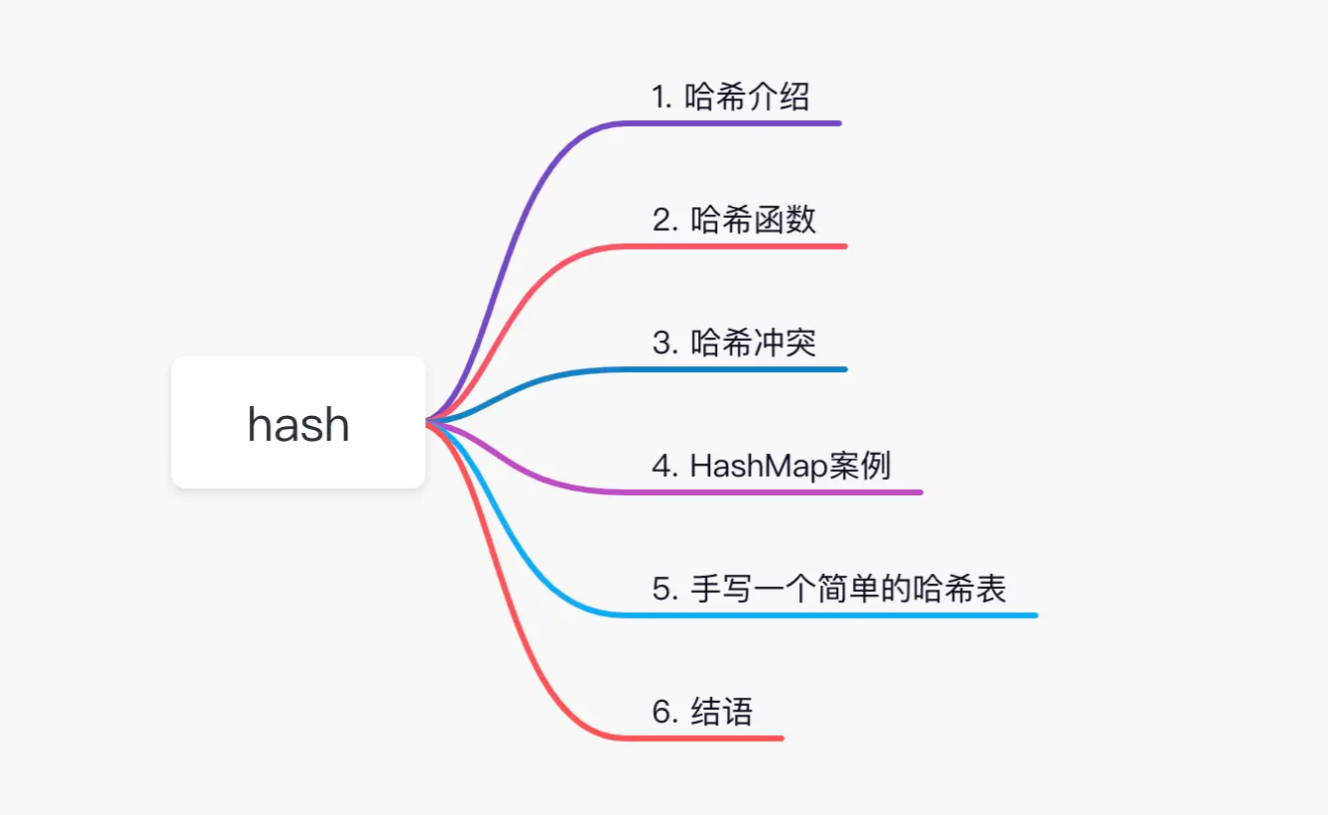## 1. 哈希表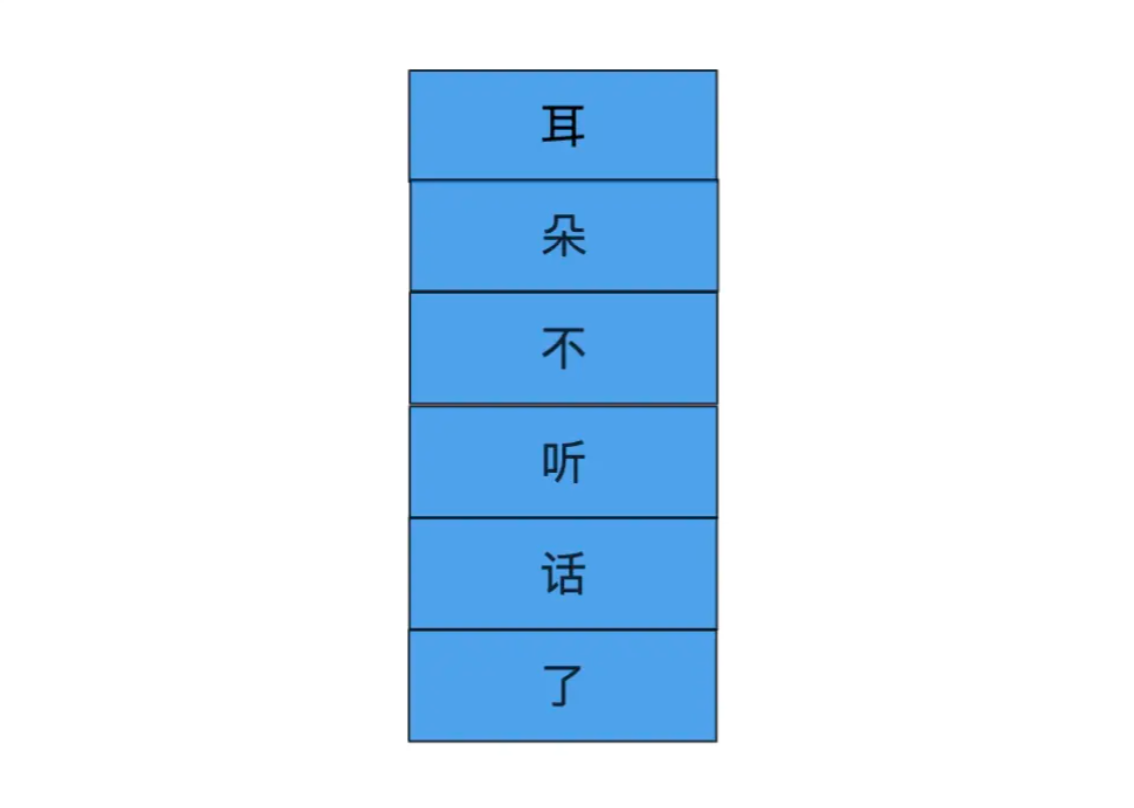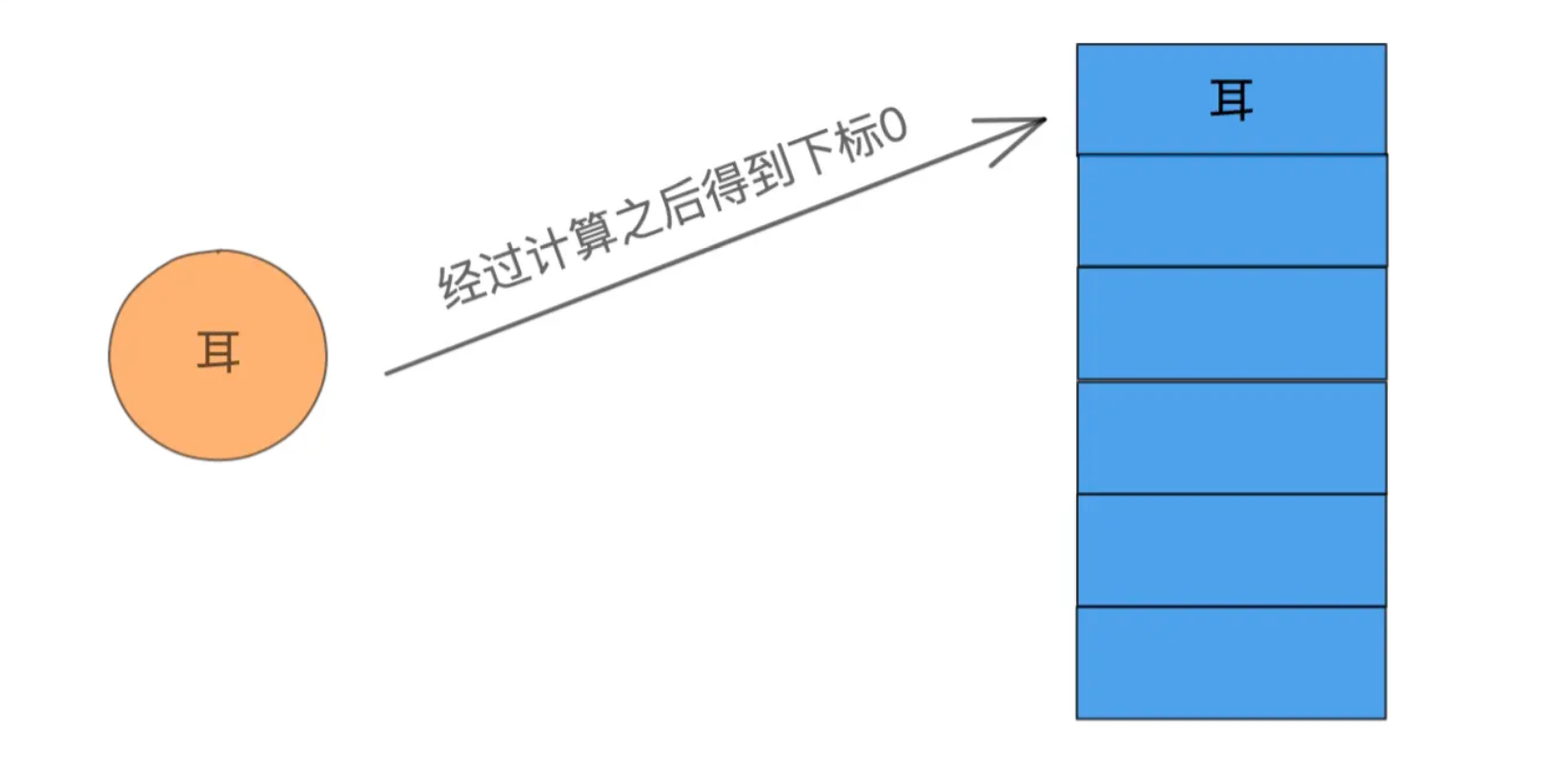1. 对键进行哈希函数计算，得到一个哈希值。

2. 对哈希值进行计算得到一个数组下标。

3. 通过数组下标得到数组中对应的数组。

## 2. 哈希函数

Integer：

```    public static int hashCode(int value) {        return value;
}复制代码```

Integer的哈希函数就是直接拿到它的值。

String：

```    public int hashCode() {        int h = hash;        if (h == 0 && value.length > 0) {
hash = h = isLatin1() ? StringLatin1.hashCode(value)
: StringUTF16.hashCode(value);
}        return h;
}复制代码```

Double：

```    public static int hashCode(double value) {        long bits = doubleToLongBits(value);        return (int)(bits ^ (bits >>> 32));
}复制代码```

`19942986 % 11 = 8`

## 3. 哈希冲突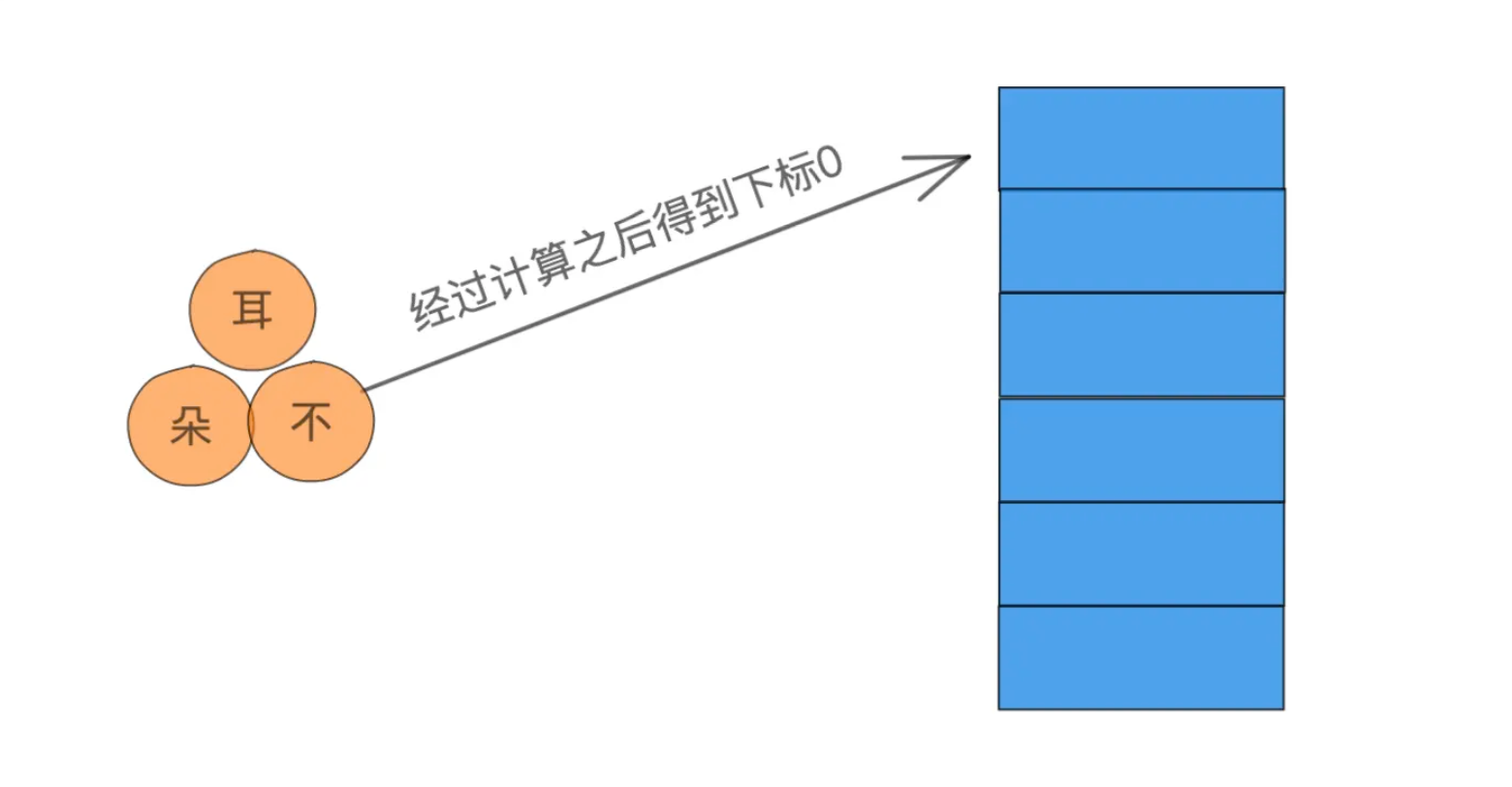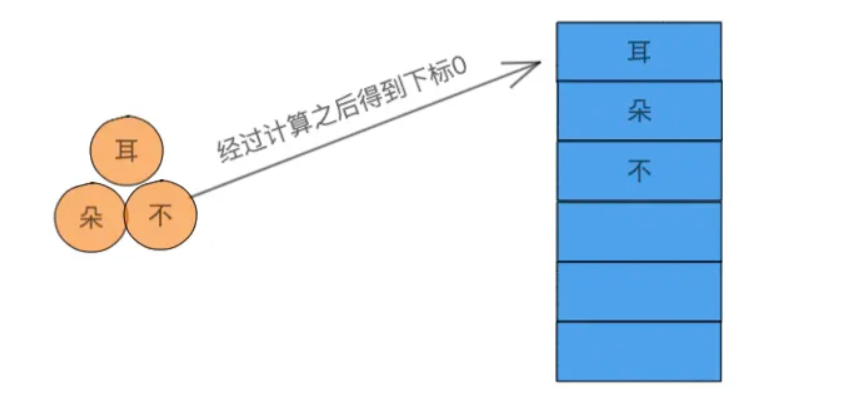1. 线性探测法：下标被占用之后，以步长为1依次向后寻找，上图例中我使用的就是这种方法。

2. 二次探测法：下标被占用之后，步长为2的倍数，依次向后寻找。

3. 伪随机探测法：下标被占用后，随机出一个数字，然后使用这个数字作为下标进行寻找，这种方法全靠天意了。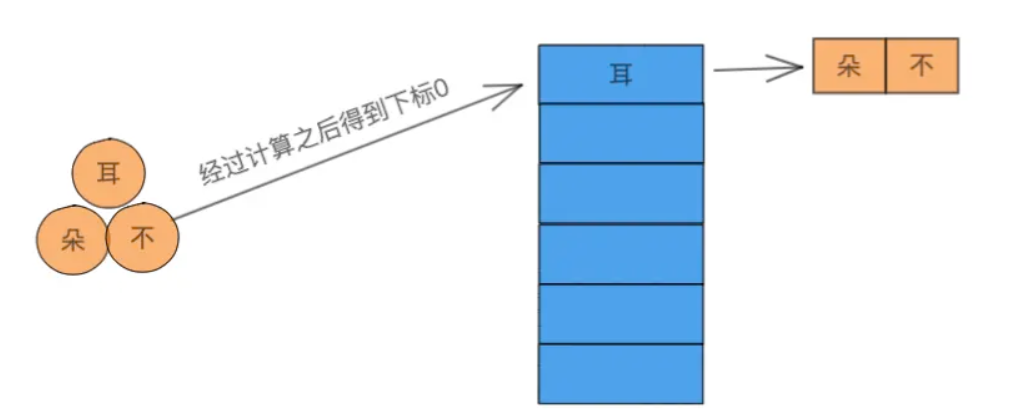## 4. HashMap案例

HashMap之所以没有使用质数而是使用2的倍数作为它的容量，是因为它在计算数组下标时没有使用除留余数法，而是使用了位运算进行计算数组下标。

```    static final int hash(Object key) {        int h;        return (key == null) ? 0 : (h = key.hashCode()) ^ (h >>> 16);
}复制代码```

## 5. 手写哈希表

1. 哈希函数

2. 哈希冲突方法

```public class DiyHashMap{    private Object[] tables;    private int size;    public DiyHashMap() {
size = 11;
tables = new Object[size];
}    public Object get(String key) {        int index = hash(key) % size;        if (tables[index] == null) {            return null;
} else {            return tables[index];
}
}    public void put(String key, Object obj) {        int index = hash(key) % size;
tables[index] = obj;
}    private int hash(String key) {        int hash = 0;        for (int i = 0; i < key.length(); i++) {
hash = hash + (key.charAt(i) * 31 + i);
}        return hash;
}

}复制代码```

1. 表可以进行扩容，扩容大小可以为原来的两倍并+1，尽可能使其是质数。

2. 使用拉链法解决冲突，我目前的做法是直接覆盖，使用拉链法可以将表的Object数组换成别的抽象数据类型数组，并多加一个双向链表引用即可。

## 6. 结语

1. 哈希冲突太多会导致部分查找线性。

2. 不能进行范围查找。

#转载请注明出处！